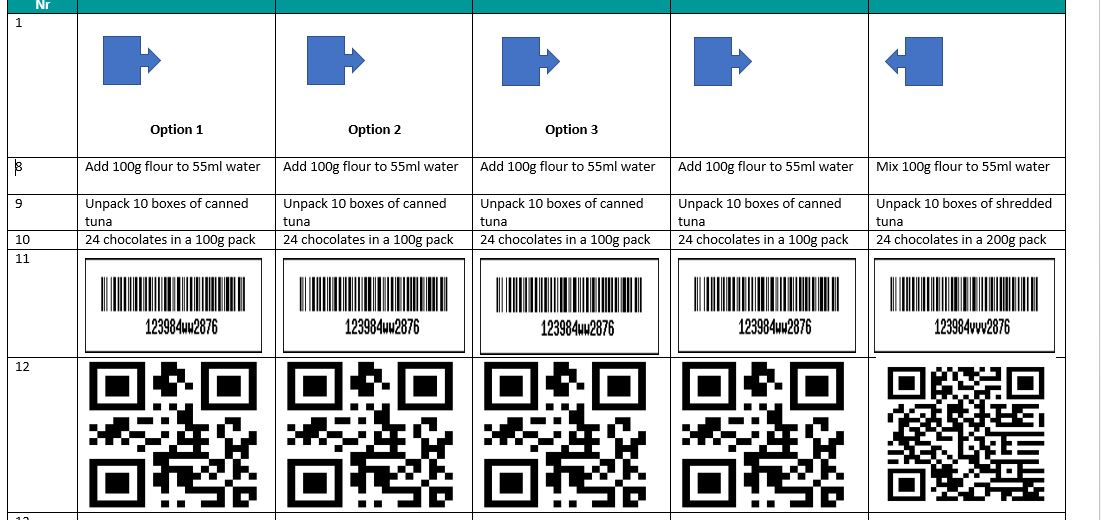# How to perform calculations using the Image Picker widget.

•martin28
Asked on September 17, 2017 at 11:52 AM

good day

What would be the easiest way to set up a survey with images like those type of questions? An image picker would of been ideal. But need to attach values to the answers. I have inquired before and no values can be added to widgets.

So the questions need to test attention to detail where participants must select the image that does not fit. A value needs to be added and a score calculated based on answers.

Your assistance and suggestions would be greatly appreciated.

Thankscalculation values   image picker   widget EditMike_G
Answered on September 17, 2017 at 06:43 AM

I see that you have opened this thread before asking if there is a way to assign calculation values to widget — https://www.jotform.com/answers/1243743

I believe there is a workaround that would meet your requirements and I can try to create a sample form for you. However, I just need the following information.

1. What is the max selection that can be selected on the Image Picker?

2. Do you need to compute the total value of the image selected per Image Picker widget? Or, do you need the total value for all the Image Picker in the form?

3. What value should each image have?

We will wait for your response.martin28
Answered on September 17, 2017 at 08:04 AM

Hi

Information you requested :

1.) The max selection is only a single answer. So only one image needs to be selected.

2. ) I will need the total value of all the image picker in the form.
The values would look like this :

Question 1 :

Image A = 0
Image B = 1 (Correct image)
Image C = 0
Image D = 0
Image E = 0

Question 2 :

Image A = 1 (Correct image)
Image B = 0
Image C = 0
Image D = 0
Image E = 0

Form calculation = Question 1 + Question 2 = Total

3. ) All questions will only have one correct answer. Thus values will only be 0's and 1's.

Thanks

•BDAVID
Answered on September 17, 2017 at 02:01 PM

1) Once you setup you images, simply add Form Calculation fields to obtain the value of the correct image, and the total:2) Identify the file name at the end of the URL for each "Correct" image:3) Then create conditions to input the value "1" in the Form Calculation widgets(which I named as 'Value from question x') if the correct image is selected: https://www.jotform.com/help/268-Conditional-Logic-Inserts-Text-Calculation-Into-A-Field4) Finally perform the calculation to get the total: https://www.jotform.com/help/259-How-to-Perform-Form-Calculation-Using-a-WidgetPlease test my demo form: https://form.jotformpro.com/72594902480966